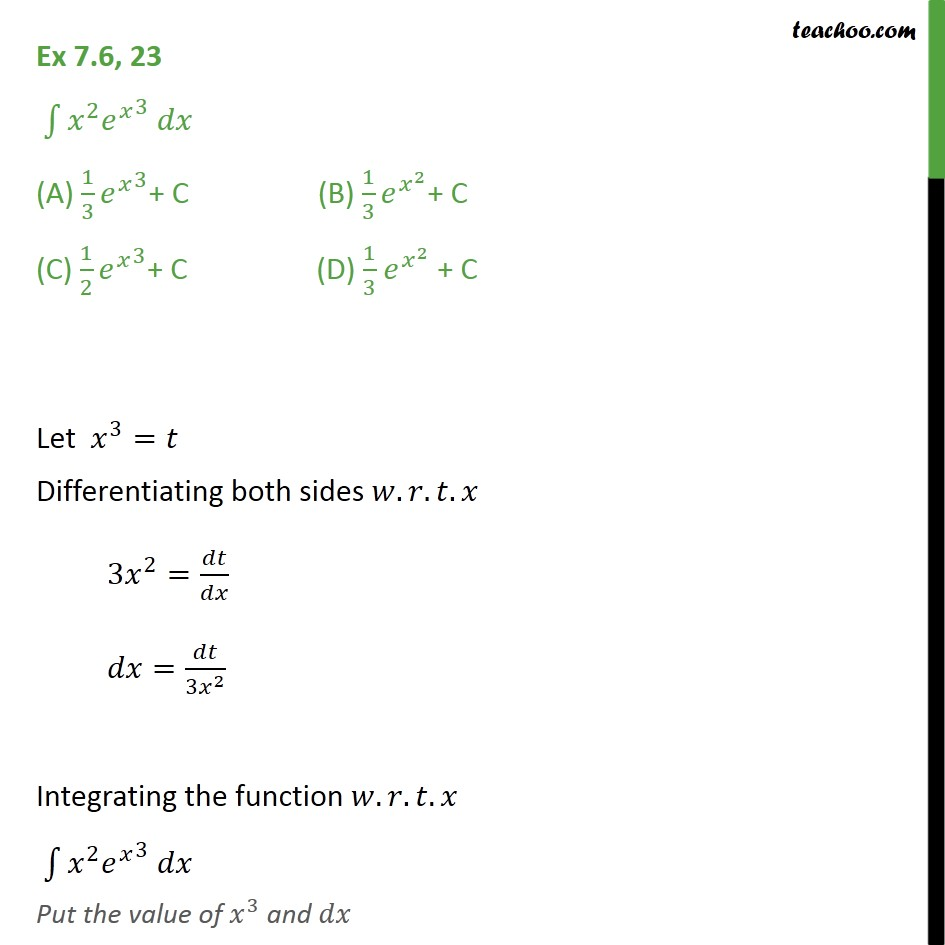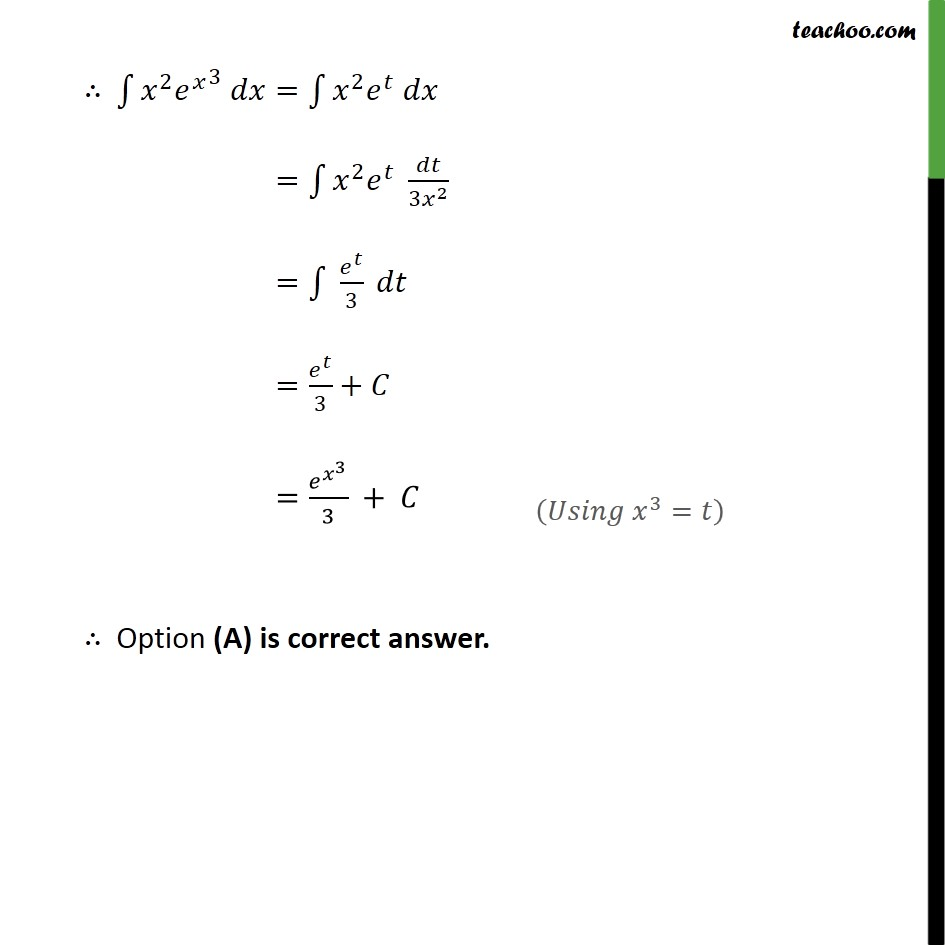Ex 7.6

Chapter 7 Class 12 Integrals (Term 2)
Serial order wise### Transcript

Ex 7.6, 23 2 3 (A) 1 3 3 + C (B) 1 3 2 + C (C) 1 2 3 + C (D) 1 3 2 + C Let 3 = Differentiating both sides . . . 3 2 = = 3 2 Integrating the function . . . 2 3 Put the value of 3 and 2 3 = 2 = 2 3 2 = 3 = 3 + = 3 3 + Option (A) is correct answer.